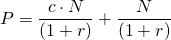# Floating rate bond

In contrast to fixed rate bonds, floating rate bonds pay coupons which vary over their maturity. The variable coupon rate is determined periodically, e.g. yearly, quarterly, … – on a fixed date that is determined by the contract.

## Floating rate bond valuation

The valuation of a floating rate bond does, at a first glance, look more complicated than that of its fixed rate counterpart. In reality, the valuation of a floating rate bond is in fact much easier. The trick lies in the fact that we should consider the principal N, as being redeemed together with the first coupon payment (which is c times N) and using the same discount rate (r). This is because both the future discount rate – not known today – as well as the coupon of the floating rate bond will be determined by the same prevailing market rate in the future.

Intuitively, interest rate increases are beneficial since the coupon increases but have at the same time also an impact on the discount rates. The opposite holds when interest rates decline. Magically, the benefits and downside of interest rate changes cancel out each other – as shown in the Excel file provided below. This explains why the principal can be considered as if it is reimbursed at the time of the next coupon payment.## Interest rate risk

Intuitively, it is already clear that interest rate risk – i.e. the risk that the bond value changes a lot when interest rates shift – is very limited for this type of bonds. This is due to the bonds’ floating nature. This is further indicated by the way floating rate bonds are valued, as if the principle is redeemed on the next coupon payment. Obviously, a bond with only 1 coupon payment including principal left has a very small duration or interest rate sensitivity.

## Floating rate instruments

When investors wish to invest in floating rate bonds, there are several approaches they can consider. First, they can invest in floating rate bond mutual funds. However, these may be subject to relatively high fees. Second, investors can opt for floating rate ETFs. Floating rate bond ETFs typically have lower total expense ratios and might thus be a more cost-effective way to invest in floating rate instruments.

Finally there’s also other financial products that are similar to floating rate bonds. For example, there also exist floating rate notes. Floating rate notes (e.g us treasury floating rate notes) can also be valued using the above formulas.

## Summary

Floating rate bonds are bonds that pay a variable coupon, depending on the prevalent market conditions at future points in time. The interest rate sensitivity of such a bond is very limited. But this comes a cost, since we are uncertain about the size of the future coupon payments.

### Floating rate bond Excel implementation

Want to value a floating rate bond? The following Excel file implements the above formulate to value such a bond: floating rate bond valuation.